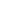# The ratio between ages of A and B is 2:3 and that between B and C is 1:2. If the sum of the ages of three is 110 years, how old is C?

a) 30
b) 40
c) 50
d) 60
KPSC Divisional Accountant-15

 60. Solution Let ages of A,B and C be x,y and z respectively. x:y = 2:3 y:z = 1:2 x = 2y/3 z = 2x Sum of three ages = x+y+Z = 110 2y/3 + y + 2x = 110 => y = 30 z = 60
Share :
+
Next
« Prev
Prev
Next »